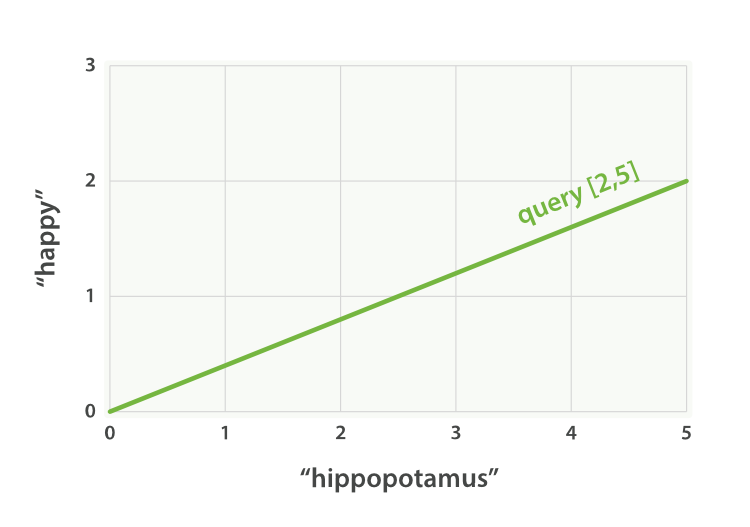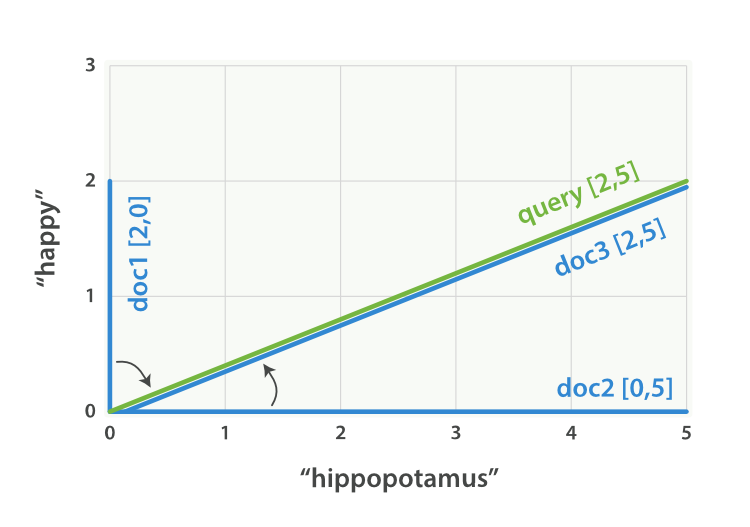## Theory Behind Relevance Scoringedit

Lucene (and thus Elasticsearch) uses the Boolean model to find matching documents, and a formula called the practical scoring function to calculate relevance. This formula borrows concepts from term frequency/inverse document frequency and the vector space model but adds more-modern features like a coordination factor, field length normalization, and term or query clause boosting.

Don’t be alarmed! These concepts are not as complicated as the names make them appear. While this section mentions algorithms, formulae, and mathematical models, it is intended for consumption by mere humans. Understanding the algorithms themselves is not as important as understanding the factors that influence the outcome.

### Boolean Modeledit

The Boolean model simply applies the `AND`, `OR`, and `NOT` conditions expressed in the query to find all the documents that match. A query for

`full AND text AND search AND (elasticsearch OR lucene)`

will include only documents that contain all of the terms `full`, `text`, and `search`, and either `elasticsearch` or `lucene`.

This process is simple and fast. It is used to exclude any documents that cannot possibly match the query.

### Term Frequency/Inverse Document Frequency (TF/IDF)edit

Once we have a list of matching documents, they need to be ranked by relevance. Not all documents will contain all the terms, and some terms are more important than others. The relevance score of the whole document depends (in part) on the weight of each query term that appears in that document.

The weight of a term is determined by three factors, which we already introduced in What Is Relevance?. The formulae are included for interest’s sake, but you are not required to remember them.

#### Term frequencyedit

How often does the term appear in this document? The more often, the higher the weight. A field containing five mentions of the same term is more likely to be relevant than a field containing just one mention. The term frequency is calculated as follows:

`tf(t in d) = √frequency `
 The term frequency (`tf`) for term `t` in document `d` is the square root of the number of times the term appears in the document.

If you don’t care about how often a term appears in a field, and all you care about is that the term is present, then you can disable term frequencies in the field mapping:

```PUT /my_index
{
"mappings": {
"doc": {
"properties": {
"text": {
"type":          "string",
"index_options": "docs"
}
}
}
}
}```
 Setting `index_options` to `docs` will disable term frequencies and term positions. A field with this mapping will not count how many times a term appears, and will not be usable for phrase or proximity queries. Exact-value `not_analyzed` string fields use this setting by default.

#### Inverse document frequencyedit

How often does the term appear in all documents in the collection? The more often, the lower the weight. Common terms like `and` or `the` contribute little to relevance, as they appear in most documents, while uncommon terms like `elastic` or `hippopotamus` help us zoom in on the most interesting documents. The inverse document frequency is calculated as follows:

`idf(t) = 1 + log ( numDocs / (docFreq + 1)) `
 The inverse document frequency (`idf`) of term `t` is the logarithm of the number of documents in the index, divided by the number of documents that contain the term.

#### Field-length normedit

How long is the field? The shorter the field, the higher the weight. If a term appears in a short field, such as a `title` field, it is more likely that the content of that field is about the term than if the same term appears in a much bigger `body` field. The field length norm is calculated as follows:

`norm(d) = 1 / √numTerms `
 The field-length norm (`norm`) is the inverse square root of the number of terms in the field.

While the field-length norm is important for full-text search, many other fields don’t need norms. Norms consume approximately 1 byte per `string` field per document in the index, whether or not a document contains the field. Exact-value `not_analyzed` string fields have norms disabled by default, but you can use the field mapping to disable norms on `analyzed` fields as well:

```PUT /my_index
{
"mappings": {
"doc": {
"properties": {
"text": {
"type": "string",
"norms": { "enabled": false }
}
}
}
}
}```
 This field will not take the field-length norm into account. A long field and a short field will be scored as if they were the same length.

For use cases such as logging, norms are not useful. All you care about is whether a field contains a particular error code or a particular browser identifier. The length of the field does not affect the outcome. Disabling norms can save a significant amount of memory.

#### Putting it togetheredit

These three factors—​term frequency, inverse document frequency, and field-length norm—​are calculated and stored at index time. Together, they are used to calculate the weight of a single term in a particular document.

When we refer to documents in the preceding formulae, we are actually talking about a field within a document. Each field has its own inverted index and thus, for TF/IDF purposes, the value of the field is the value of the document.

When we run a simple `term` query with `explain` set to `true` (see Understanding the Score), you will see that the only factors involved in calculating the score are the ones explained in the preceding sections:

```PUT /my_index/doc/1
{ "text" : "quick brown fox" }

GET /my_index/doc/_search?explain
{
"query": {
"term": {
"text": "fox"
}
}
}```

The (abbreviated) `explanation` from the preceding request is as follows:

```weight(text:fox in 0) [PerFieldSimilarity]:  0.15342641
result of:
fieldWeight in 0                         0.15342641
product of:
tf(freq=1.0), with freq of 1:        1.0
idf(docFreq=1, maxDocs=1):           0.30685282
fieldNorm(doc=0):                    0.5 ```
 The final `score` for term `fox` in field `text` in the document with internal Lucene doc ID `0`. The term `fox` appears once in the `text` field in this document. The inverse document frequency of `fox` in the `text` field in all documents in this index. The field-length normalization factor for this field.

Of course, queries usually consist of more than one term, so we need a way of combining the weights of multiple terms. For this, we turn to the vector space model.

### Vector Space Modeledit

The vector space model provides a way of comparing a multiterm query against a document. The output is a single score that represents how well the document matches the query. In order to do this, the model represents both the document and the query as vectors.

A vector is really just a one-dimensional array containing numbers, for example:

`[1,2,5,22,3,8]`

In the vector space model, each number in the vector is the weight of a term, as calculated with term frequency/inverse document frequency.

While TF/IDF is the default way of calculating term weights for the vector space model, it is not the only way. Other models like Okapi-BM25 exist and are available in Elasticsearch. TF/IDF is the default because it is a simple, efficient algorithm that produces high-quality search results and has stood the test of time.

Imagine that we have a query for “happy hippopotamus.” A common word like `happy` will have a low weight, while an uncommon term like `hippopotamus` will have a high weight. Let’s assume that `happy` has a weight of 2 and `hippopotamus` has a weight of 5. We can plot this simple two-dimensional vector—`[2,5]`—as a line on a graph starting at point (0,0) and ending at point (2,5), as shown in Figure 27, “A two-dimensional query vector for “happy hippopotamus” represented”.Figure 27. A two-dimensional query vector for “happy hippopotamus” represented

Now, imagine we have three documents:

1. I am happy in summer.
2. After Christmas I’m a hippopotamus.
3. The happy hippopotamus helped Harry.

We can create a similar vector for each document, consisting of the weight of each query term—`happy` and `hippopotamus`—that appears in the document, and plot these vectors on the same graph, as shown in Figure 28, “Query and document vectors for “happy hippopotamus””:

• Document 1: `(happy,____________)``[2,0]`
• Document 2: `( ___ ,hippopotamus)``[0,5]`
• Document 3: `(happy,hippopotamus)``[2,5]`Figure 28. Query and document vectors for “happy hippopotamus”

The nice thing about vectors is that they can be compared. By measuring the angle between the query vector and the document vector, it is possible to assign a relevance score to each document. The angle between document 1 and the query is large, so it is of low relevance. Document 2 is closer to the query, meaning that it is reasonably relevant, and document 3 is a perfect match.

In practice, only two-dimensional vectors (queries with two terms) can be plotted easily on a graph. Fortunately, linear algebra—the branch of mathematics that deals with vectors—​provides tools to compare the angle between multidimensional vectors, which means that we can apply the same principles explained above to queries that consist of many terms.

You can read more about how to compare two vectors by using cosine similarity.

Now that we have talked about the theoretical basis of scoring, we can move on to see how scoring is implemented in Lucene.